# GMAT Math : Calculating discrete probability

## Example Questions

← Previous 1 3 4 5 6 7 8

### Example Question #1 : Discrete Probability

Race cars for a particular race are numbered sequentially from 12 to 115. What is the probability that a car selected at random will have a tens digit of 1?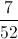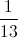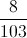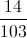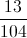Explanation:

There are 104 integers from 12 to 115 inclusive. There are 8 integers from 12 to 19 and 6 integers from 110 to 115 for a total of 14 integers with a tens digit of 1. The probability of selecting a car with a tens digit of 1 is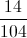=.

### Example Question #1 : Discrete Probability

Three friends play marbles each week. When they combine their marbles, they have 100 in total. 45 of the marbles are new and the rest are old. 30 are red, 20 are green, 25 are yellow, and the rest are white. What is the probability that a randomly chosen marble is new OR yellow?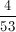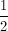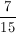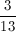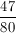Explanation:

Prob(new OR yellow) = P(new) + P(yellow) - P(new AND yellow)

Prob(new) =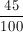Prob(yellow) =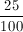Prob(new AND yellow) =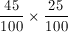so P(new OR yellow) =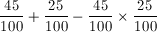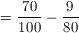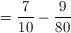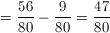### Example Question #3 : Discrete Probability

Flight A is on time for 93% of flights.  Flight B is on time for 89% of flights.  Flight A and B are both on time 87% of the time.  What is the probabiity that at least one flight is on time?

0.87

0.93

0.89

0.95

1.82

0.95

Explanation:

P(Flight A is on time) = 0.93

P(Flight B is on time) = 0.89

P(Flights A and B are on time) = 0.87

Then P(A OR B) = P(A) + P(B) - P(A AND B) = 0.93 + 0.89 – 0.87 = 0.95

### Example Question #4 : Discrete Probability

At a school fair, there are 25 water balloons.  10 are yellow, 8 are red, and 7 are green.  You try to pop the balloons.  Given that you first pop a yellow balloon, what is the probability that the next balloon you hit is also yellow?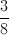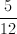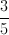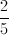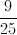Explanation:

At the start, there are 25 balloons and 10 of them are yellow.  You hit a yellow balloon.  Now there are 9 yellow balloons left out of 24 total balloons, so the probability of hitting a yellow next is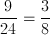.

### Example Question #5 : Discrete Probability

A bag has 7 blue balls and 3 red balls.  2 balls are to be drawn successively and without replacement.  What is the probability that the first ball is red and the second ball is blue?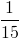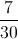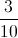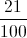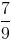Explanation:

We first have 7 blue and 3 red out of 10, so P(1st ball is red) =.  Now, we have pulled a red ball out of the bag, leaving us with 7 blue and 2 red out of 9 total balls. Then P(2nd ball is blue) =.  Put this together, P(1st red AND 2nd blue) =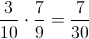.

### Example Question #6 : Discrete Probability

4 cards are to be dealt successively and without replacement from an ordinary deck of 52 cards.  What is the probability of receiving, in order, a spade, a heart, a diamond, and a club?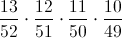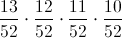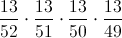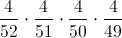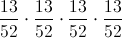Explanation:

There are 4 suits, and each suit has 13 cards, so P(spade) =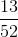Now there are 51 cards left: 12 spades, 13 hearts, 13 diamonds, and 13 clubs, so now P(heart) =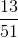Once again, there are now 50 cards: 12 spades, 12 hearts, 13 diamonds, and 13 clubs, so now P(diamond) =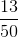Before our last draw we have 49 cards left: 12 spades, 12 hearts, 12 diamonds, and 13 clubs, so P(club) =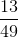Putting these four probabilities together gives us our answer:

P(spade AND heart AND diamond AND club) =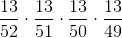### Example Question #1 : Discrete Probability

A red die and a white die are rolled.  What is the probability of getting a 4 on the red die AND an odd sum of numbers on the two dice?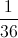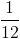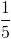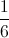Explanation:

Let's first look at the two probabilities separately.

There are 36 possible combinations of the two dice: (1, 1), (1, 2), (1, 3),...,(1, 6); (2, 1), (2, 2), (2, 3),...; ....; (6, 1), ..., (6, 6).  Getting a 4 on the red die can happen 6 different ways: (4, 1), (4, 2), (4, 3), (4, 4), (4, 5), and (4, 6). So P(4 on red die)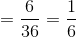.

Now, getting an odd sum on the dice can happen 18 different ways: (1, 2), (1, 4), (1, 6), (2, 1), (2, 3), (2, 5), (3, 2), (3, 4), (3, 6), (4, 1), (4, 3), (4, 5), (5, 2), (5, 4), (5, 6), (6, 1), (6, 3), and (6, 5).  So P(odd sum) =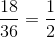.

Putting them together, P(4 on red AND odd sum) =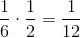.

### Example Question #8 : Discrete Probability

What is the probability of sequentially drawing 2 diamonds from a deck of regular playing cards when the first card is not replaced?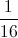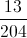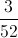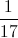Explanation:

The probability of drawing a diamond first is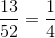The probabilty of drawing a diamond second when the first card is not replaced is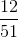.

To determine the probability of 2 INDEPENDENT events, we multiply them.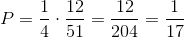### Example Question #9 : Discrete Probability

Two fair dice are tossed. What is the probability that the dice will add up to a prime number?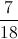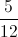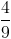Explanation:

The prime numbers that can be rolled are 2, 3, 5, 7, and 11. The rolls that result in one of these numbers, out of a possible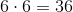rolls, are: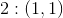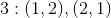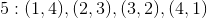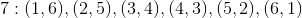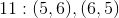Therefore, there are 15 rolls out of 36 that result in a prime number, making the probability of a prime result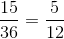### Example Question #10 : Discrete Probability

Two fair six-sided dice are rolled. What is the probability that the sum is either a perfect square or a perfect cube?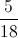Explanation:

There are 36 possible rolls. The only perfect squares that can be rolled are 4 and 9; the only perfect cube that can be rolled is 8. So the roll must be one of the following: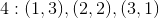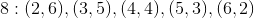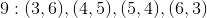This adds up to 12 rolls out of 36, for a probability of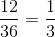← Previous 1 3 4 5 6 7 8

Tired of practice problems?

Try live online GMAT prep today.